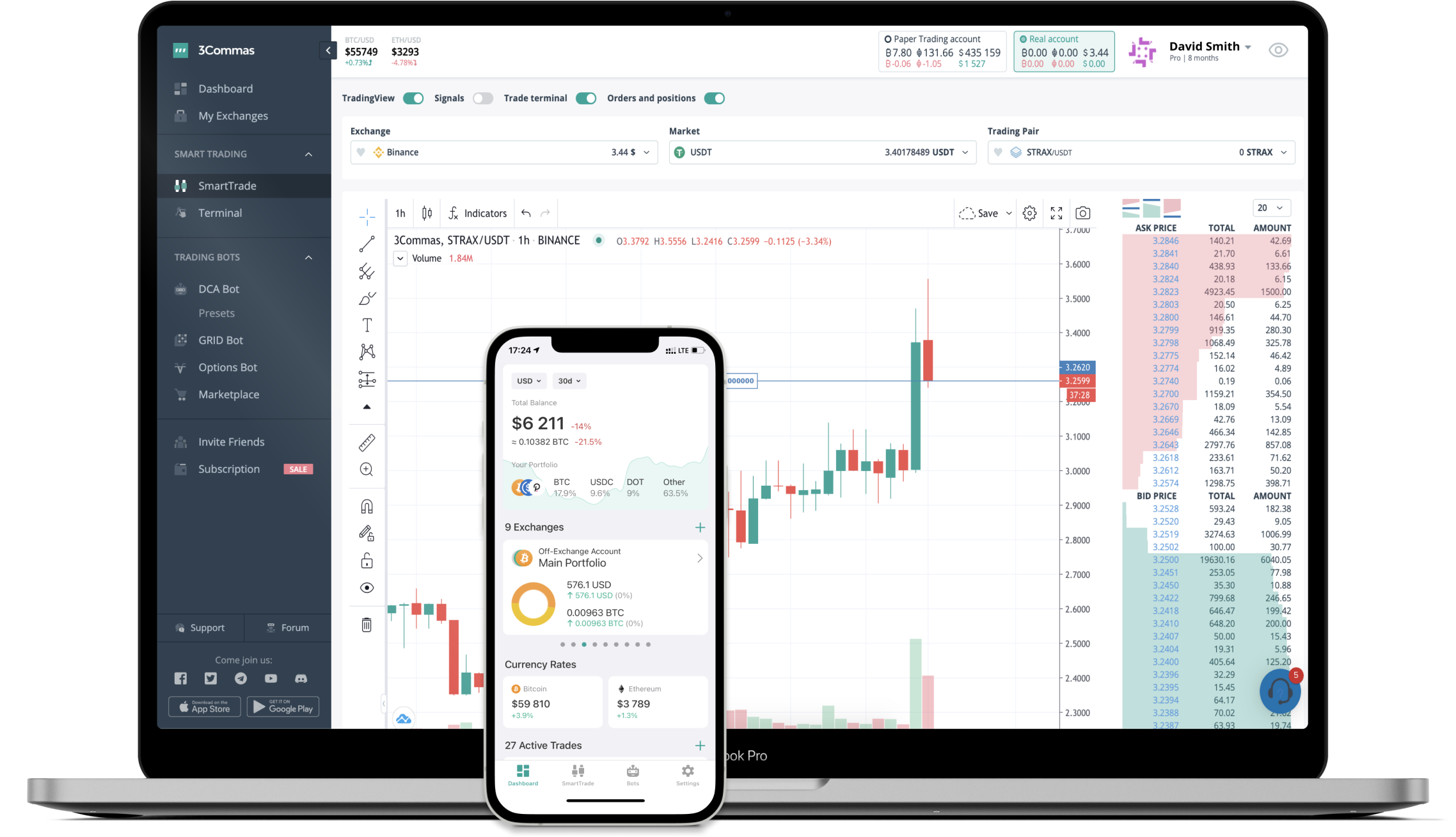# EQUAL to AUD Converter

Equalizer DEX
PriceAUD 10.94+2.28%
VolumeAUD 204,256
How it work

The 3Commas currency calculator allows you to convert a currency from Equalizer DEX (EQUAL) to Australian Dollar (AUD) in just a few clicks at live exchange rates.

Simply enter the amount of Equalizer DEX you wish to convert to AUD and the conversion amount automatically populates. You can also use our Prices Calculator Table to calculate how much your currency is worth in other denominations, i.e. .1 EQUAL, .5 EQUAL, 1 EQUAL, 5 EQUAL, or even 10 EQUAL.

## EQUAL to AUD Exchange Calculator

The chart displays Equalizer DEX price changes in AUD.

The ultimate tools for crypto traders to maximize trading profits while minimizing risk and loss.## Latest EQUAL to AUD price calculator

Equalizer DEX market price is updated every three minutes and is automatically displayed in AUD. Below are the most popular denominations to convert to AUD.

Equalizer DEX
Australian Dollar
0.11
AUD
1.09
AUD
10.94
AUD
21.88
AUD
32.82
AUD
54.70
AUD
109.40
AUD
273.50
AUD
547.00
AUD
1094.00
AUD
2735.00
AUD
5470.00
AUD
10940.00
AUD
27350.00
AUD
Australian Dollar
Equalizer DEX
0.00091408
EQUAL
0.00914077
EQUAL
0.09140768
EQUAL
0.18281536
EQUAL
0.27422303
EQUAL
0.45703839
EQUAL
0.91407678
EQUAL
2.28519196
EQUAL
4.57038391
EQUAL
9.14076782
EQUAL
22.85191956
EQUAL
45.70383912
EQUAL
91.40767824
EQUAL
228.51919561
EQUAL

## Top Currencies